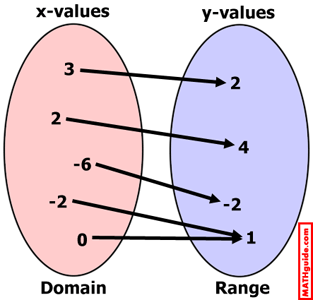Relations
 Home > Lessons > Relations Search | Updated October 27th, 2016
Introduction

Within this MATHguide page, you will learn various characteristics of relations.

 What is a Relation? A relation is a set of ordered pairs. For instance, set S below is a relation.     S = {(3,2), (2,4), (-6,-2), (-2,1), (0,1)}     Practically speaking, relations are a comparison from one set to another. For instance, people often look at their earnings over time. We connect the years we have been employed to the earnings we make that year.     Someone else may be shopping for an above ground pool. That person may want to compare the size of the pool (diameter) to the cost of maintaining it. This would be very useful for someone who is on a tight budget but also wants easy access to a pool. Mapping Relations Sometimes relations are viewed as a connection of elements from one set to the elements of another set. Using set S from our last section, this diagram will visually indicate what a mapping of sets looks like.Notice a few things from the mapping above. It allows us to see that the set of all x-values has a special name, as does the set of all y-values. Also, we can see that two of the x-values map to an identical y-value: referring to (-2,1) and (0,1). This has special meaning and it will be explained in greater detail in the following sections within this page. Domain and Range We have a special page dedicated to domain and range. You can access it by using the link below.esson: Domain and Range Inverses Several sections above, we used set S. This is set S.     S = {(3,2), (2,4), (-6,-2), (-2,1), (0,1)} To construct the inverse of set S, simply switch the x-values and the y-values, like so.     S-1 = {(2,3), (4,2), (-2,-6), (1,-2), (1,0)}     Notice that the inverse of set S is written as S-1.     If we use function notation, the inverse of f(x) is written as f-1(x).     We have a special page dedicated to inverses because there is more to the story. You can access this page by using the link below.esson: Inverses Functions Simply put, a function is a certain type of relation. To learn about functions, use the link below.esson: Functions Polynomials Polynomials are special types of functions. To learn about polynomials, use the link below.esson: Polynomials Related Lessons Try this lesson, which is closely related to the lesson above.esson: Domain and Rangeesson: Inversesesson: Functionsesson: Translationsesson: Testing Relations for Symmetryesson: Operations on Polynomialsesson: Linear Programming